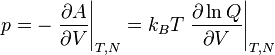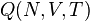# Difference between revisions of "Pressure"

Pressure ($p$) is the force per unit area applied on a surface, in a direction perpendicular to that surface, i.e. the scalar part of the stress tensor. In thermodynamics the pressure is given by$p = - \left.\frac{\partial A}{\partial V} \right\vert_{T,N} = k_BT \left.\frac{\partial \ln Q}{\partial V} \right\vert_{T,N}$

where$A$ is the Helmholtz energy function,$V$ is the volume,$k_B$ is the Boltzmann constant,$T$ is the temperature and$Q (N,V,T)$ is the canonical ensemble partition function.

## Units

The SI units for pressure are Pascals (Pa), 1 Pa being 1N/m$^2$, or 1J/m$^3$. Other usual units are bars and millibars (mbar); 1mbar=100Pa=1hPa, 1 hectopascal. 1 bar is$10^5$Pa by definition. This is very close to the standard atmosphere (atm), approximately equal to typical air pressure at earth mean sea level:

atm, standard atmosphere = 101325 Pa = 101.325 kPa = 1013.25 hPa = 1.01325 bar